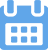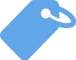# Standard Deviation Meaning: Here’s What It Means and How to Use It

•Kevin
•September 15, 2023
•### Your writing, at its best

Compose bold, clear, mistake-free, writing with Grammarly's AI-powered writing assistant

If you’ve ever taken a statistics class, you may be familiar with the term standard deviation (ˌstæn.dɚd ˌdiː.viˈeɪ.ʃən), but do you know what it means or how it’s calculated? Not to worry—we’re here to help!

In this post, we’re diving into the world of mathematics to uncover the definition behind standard deviation, its usage, and more. So, if you’ve ever wondered about our word of the day, keep reading!

## What Is the Definition of Standard Deviation?

According to the Collins English Language Dictionary, standard deviation (typically denoted by σ) is a statistical measurement. That said, the standard deviation formula is as follows:

s=√1n−1∑ni=1(xi−¯x)2s=1n−1∑i=1n(xi−x¯)2.

This statistic measures the dispersion of a data set relative to its mean (or average) and is then calculated by finding the square root of the variance.

• Standard deviation is a statistic used mostly in the finance realm. For example, a stable blue-chip stock most commonly has a low standard deviation, with a volatile stock traditionally having a higher standard deviation.
• Concerning finance, standard deviation is often used to measure the relative risk involved with a certain asset or stock.
• In layman’s terms, standard deviation measures the dispersion of a dataset relative to its average.

### How Can I Calculate Standard Deviation?

The standard deviation is usually calculated automatically, but you can also calculate it by hand to better understand how the formula works.

That said, there are six main steps for finding the standard deviation when calculating by hand:

• Step 1: Find the mean. To identify the mean, simply add up all the scores before dividing them by the number of scores.
• Step 2: Find each score’s deviation from the mean number. Subtract the mean from each score to identify the deviations from the mean.
• Step 3: Square each deviation from the mean. Next, multiply each deviation from the mean. This will result in positive numbers.
• Step 4: Find the sum of squares. Add up all the squared deviations (AKA the sum of squares).
• Step 5: Locate the variance. Divide the sum of squares by either N (for population data) or (n – 1) for sample data.
• Step 6: Discover the square root of the variance. Take the square root of the result from step 5 to discover the standard deviation.

## What Are Synonyms and Antonyms of Standard Deviation?

Now that you’re better acquainted with the term standard deviation, it’s time to review some synonyms and antonyms. In short, a synonym is a word or phrase with the same — or nearly the same — meaning as another word.

On the other hand, an antonym is a wordor phrase that has the opposite meaning of another word or phrase.

Below you will find a few antonyms and synonyms of standard deviation provided by Power Thesaurus:

### Synonyms

• Deviation
• Normal deviation
• Predictable error
• Stat
• Probable error
• Range of error
• SD
• Standard Error
• Sigma

### Antonyms

• Random error
• Accidental blunder
• Accidental error
• Arbitrary error
• Exceptional mistake
• Casual error
• Chance error

## What Are Translations of Standard Deviation?

Wondering how to say our word of the day in a different language? We’ve got you covered! Here are some translations of standard deviation found all around the globe:

• Arabic — الانحراف المعياري
• Danish — standardafvigelse
• Korean — 표준 편차
• Norwegian — standardavvik
• Polish — odchylenie standardowe
• Russian — стандартное отклонение
• Dutch — standaarddeviatie
• Finnish — keskihajonta
• Bulgarian — стандартно отклонение
• Chinese (simplified) — 标准差
• Croatian — standardna devijacija
• French — écart type
• German — Standardabweichung
• Spanish — desviación estándar
• Turkish — standart sapma
• Ukrainian — стандартне відхилення
• Vietnamese — độ lệch chuẩn
• Swedish — standardavvikelse
• Thai — ส่วนเบี่ยงเบนมาตรฐาน
• Italian — deviazione standard
• Japanese — 標準偏差
• Afrikaans — standaardafwijking
• Greek — τυπική απόκλιση
• Czech — směrodatná odchylka

### Related Terms You Should Know

To further your overall understanding of standard deviation, we suggest reviewing the related terms below:

• Variance — the expectation of the squared deviation of a random variable from its population mean or sample mean
• Data points — a piece of information that describes one unit of observation
• Normal distribution — a tool to understand standard deviation (AKA the bell curve)
• Sample standard deviation — the root-mean-square of the differences between observations and the sample mean
• Population standard deviation — a parameter, which is a fixed value calculated from every individual in the population
• High standard deviation — a measure that indicates that the values are spread out over a wider range
• Low standard deviation — a measure that indicates that the values tend to be close to the mean (AKA the expected value)
• Data values — the content that fills a space in a record
• Absolute value — a mathematical term used to indicate the distance point or number from the origin (AKA zero point) of a number line or coordinate system
• Measure of dispersion — a way of describing how spread out a set of data is
• Sample variance — used to calculate how varied a sample is

## Conclusion

Our word of the day can be defined as a statistical measurement primarily used in finance.

Standard deviation, which is found by calculating the square root of the variance, is then applied to the annual rate of return of an investment and used to shed light on that particular investment’s historic volatility.

The standard deviation is essential because it tells us how spread out the values are in a given dataset (AKA, a set or collection of data).

Whenever someone analyzes a dataset, they are interested in finding the following metrics: The center of the dataset. The most common way to measure the “center” is with the mean and the median.

Sources:

Standard deviation definition and meaning | Collins English Dictionary

Standard Deviation: Standard Deviation | National Library of Medicine